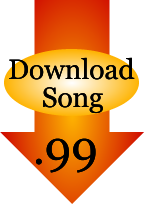# 50 States

"Let's Learn the 50 States" Memory Tester Lyrics

(Hint: You can see the answer if you hold your mouse over the blank)

Let's learn the 50 states
You can learn the 50 states
Let's learn the 50 states
You can learn the 50 states
Let's start the song - with A through D
1, 2, 3...

A_____________ and A_____________,
A_____________, A_____________
C_____________, C_____________
C_____________ and that's not all

Where, Oh where is D_____________?
(It's on the East Coast; it was our first state!)
Now you've got the first eight!

F_____________'s the next state
Then G_____________ and H_____________

Add 2 K's with K_____________ and K_____________
But just 1 "L"... that L_____________'s lucky

Let's learn the 50 states
You can learn the 50 states

M_____________, M_____________, M_____________
If you know the next 4 you get a banana
M_____________, M_____________, M_____________, M_____________

Half way done and I'm really proud of ya
On to the "N's": N_____________, N_____________

4 states with "New" forget them you're a dork
New H_____________, New J_____________, New M_____________, New Y_____________,
North C_____________, North D_____________, let's sing the chorus, here we go-a

Let's learn the 50 states
(East coast and West coast)
You can learn the 50 states
(Across the Midwest)

Let's learn the 50 states
(Down in the South)
You can learn the 50 states
(and way up North)

Ohio, Oklahoma, Oregon way to go!

A B C D E F G H I J K L M N O P_____________,
Q, R_____________ Island, South C_____________, South D_____________
Are you getting thirsty and wanna drink a soda?
Just 9 states on the list
Let's do in 3/4 time and you'll get the gist

T_____________, T___________, U__________
V_____________, V_____________, W_____________
3 more "W's" for you to sing
West V_____________, W_____________ and W_____________

Let's learn the 50 states
(Where do you live?)
You can learn the 50 states
(In the USA)

Let's learn the 50 states
You can learn the 50 states
(In the USA)

Let's learn the 50 states
(Where have you been?)
You can learn the 50 states
(In the USA)

Let's learn the 50 states
You can learn the 50 states
50 states!

`© 2005 Power Arts Company, Inc.`CD Baby
iTunesSongs for Teaching
CD Baby
iTunesCD Baby

Reviews

Bonus video of this song: